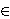### Maja Fošner and Joso Vukman

Faculty of logistics, University of Maribor, Mariborska cesta 2, 3000 Celje, Slovenia
e-mail: maja.fosner@uni-mb.si

Department of Mathematics and Computer Science, Faculty of natural sciences and mathematics, University of Maribor, Koroška cesta 160, SI-2000 Maribor, Slovenia
e-mail: joso.vukman@uni-mb.si

Dedicated to the memory of Professor Svetozar Kurepa

Abstract.   In this paper we prove the following result. Let m ≥ 0 and n ≥ 0 be integers with m+n ≠ 0 and let R be a prime ring with char(R)=0 or m+n+1 ≤ char(R) ≠ 2. Suppose there exists a nonzero additive mapping D:R → R satisfying the relation D(xm+n+1)=(m+n+1)xmD(x)xn for all xR. In this case D is a derivation and R is commutative.

2000 Mathematics Subject Classification.   16N60, 39B05.

Key words and phrases.   Prime ring, functional identity, derivation.

Full text (PDF) (access from subscribing institutions only)

DOI: 10.3336/gm.46.1.06

References:

1. K. I. Beidar, M. Brešar and M. A. Chebotar, Functional identities revised: the fractional and the strong degree, Comm. Algebra 30 (2002), 935-969.
MathSciNet     CrossRef

2. K. I. Beidar and M. A. Chebotar, On functional identities and d-free subsets of rings I, Comm. Algebra 28 (2000), 3925-3951.
MathSciNet     CrossRef

3. K. I. Beidar and Y. Fong, On additive isomorphisms of prime rings preserving polynomials, J. Algebra 217 (1999), 650-667.
MathSciNet     CrossRef

4. K. I. Beidar, W. S. Martindale, III and A. V. Mikhalev, Rings with generalized identities, Marcel Dekker, Inc., New York, 1996.
MathSciNet

5. K. I. Beidar, A. V. Mikhalev and M. A. Chebotar, Functional identities in rings and their applications, Russian Math. Surveys 59 (2004), 403-428.
MathSciNet     CrossRef

6. M. Brešar, A generalization of the notion of centralizing mappings, Proc. Amer. Math. Soc. 114 (1992), 641-649.
MathSciNet     CrossRef

7. M. Brešar, Functional identities: A survey, Contemp. Math. 259 (2000), 93-109.
MathSciNet

8. M. Brešar and J. Vukman, On left derivations and related mappings, Proc. Amer. Math. Soc. 110 (1990), 7-16.
MathSciNet     CrossRef

9. Q. Deng, On Jordan left derivations, Math. J. Okayama Univ. 34 (1992), 145-147.
MathSciNet

10. E. C. Posner, Derivations in prime rings, Proc. Amer. Math. Soc. 8 (1957), 1093-1100.
MathSciNet     CrossRef

11. L. H. Rowen, Some results on the center of a ring with polynomial identity, Bull. Amer. Math. Soc. 79 (1973), 219-223.
MathSciNet     CrossRef

12. J. Vukman, Commuting and centralizing mappings in prime rings Proc. Amer. Math. Soc. 109 (1990), 47-52.
MathSciNet     CrossRef

13. J. Vukman, On left Jordan derivations of rings and Banach algebras, Aequationes Math. 75 (2008), 260-266.
MathSciNet     CrossRef

14. J. Vukman and M. Fošner, A characterization of two-sided centralizers on prime rings, Taiwanese J. Math. 11 (2007), 1431-1441.
MathSciNet

15. J. Vukman and I. Kosi-Ulbl, On some equations related to derivations in rings, Internat. J. Math. Math. Sci. 17 (2005), 2703-2710.
MathSciNet     CrossRef